### Home > INT3 > Chapter 10 > Lesson 10.2.1 > Problem10-110

10-110.
1. Without using a calculator, solve these two problems from a college
entrance exam. Homework Help ✎1. If x–4/3 + 1 = 17, what is x–1/3?

2. If log(A) = a what is log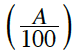?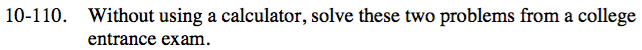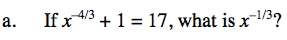Solve the first equation for x, then use that value to find the answer.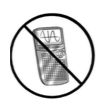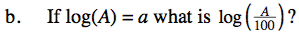a − 2